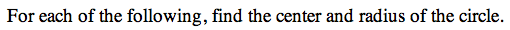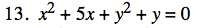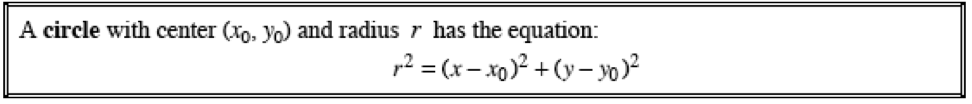13C-13.

### Missing problem for Book PCT => Chapter Ch13 => Lesson 13.1.1 => Problem 13C-13

Created from orphaned homework help problemComplete the square to put the equation in Standard Form.

(x2 + 5x + 25/4) + (y2 + y + 1/4) = 0 + 25/4 + 1/4